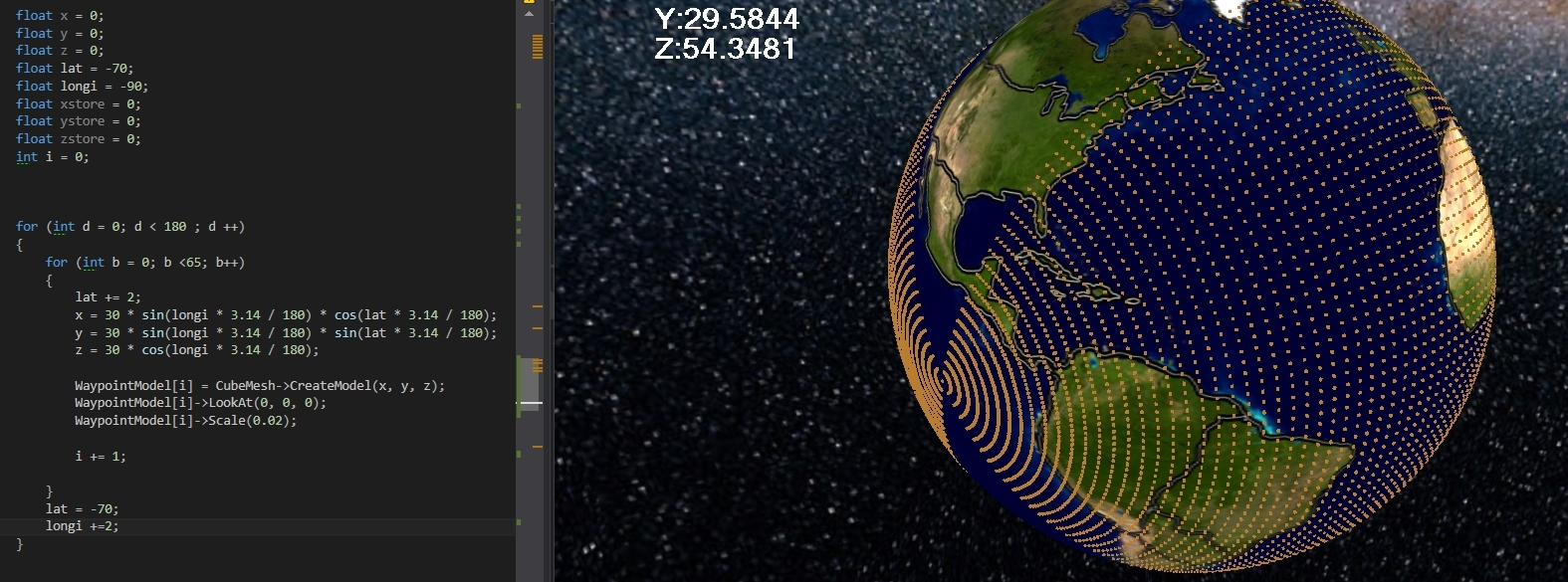# Create a series of points round a globe (Polar to Cartesian) C++

I am trying to create a series of waypoints for my globe planet, in a little simulation I'm doing, and I'm trying to use polar to cartesian coordinates, I learnt this formula off its wikipedia page. Though I still don't quite understand it.

https://en.wikipedia.org/wiki/Spherical_coordinate_system
Attempt 1; This is the image of my first attempt, I do not want to cluster the points near the poles, the poles are also at the completely wrong point, I will supply my code later on. But also I do not want to put points too close to the poles, as they are not in use, and due to camera gimbal locking, there is no point me going too far close to the north or south pole in my program.Attempt 2: Now I know how to create a single line, and the code I use for this is provided below. I sort of figured this out by just testing out different values and seeing how it came about.##Now what I want to do to this, I think as the way I wish to construct it, is to get the vector of each of those points, and rotate them round the centre of the planet which in this example is (0,0,0)I want to create a single line of longitude per pas, so I can number the points 0-50 on each line, that will allow me to use it as a connected grid,lets say 0-50 going upwards, so that if i start at point 1 which would be the first point near the south pole, and I want to go to the next row I would add 50 to get to 51 (which would be the point right of it) or take 50 to get the point left of it), and so on and so forth, this will allow me to easily join waypoints for pathfinding setup.I made another attempt in the link [ABOVE], where I try and make a nested for loop and change the latitude per step, but it does not get the desired result, I have even swapped the values of lat and longitude around in the code and the coordinates are the same.

Latitude = should be the angle from east to west. Longitude = should be the angle north to south.

To do this I think but I am not sure, I assume I will have to store a temporary value of each of those points, and then create a new vector from them, by rotating their vectors around the origin of the planet (0,0,0) and then creating a new set of these points at the temporary vectors.

Does anybody have an idea how to help me solve my problem, as Image 4 is the best explanation I can think of showing my problem to explain what I am trying to achieve.

• I'm not quite sure if I can follow what you're asking, but another term that might be helpful to search for is great-circle ark or distance. It's the shortest distance between two points on the surface of a sphere, which might have some application to pathfinding on a planet. – derstander Mar 8 '17 at 5:09
• Also helpful might be the definitions of longitude and latitude which are the lines you are describing. – user1118321 Mar 8 '17 at 5:22
• My first idea, is to take all those points as a vector, and rotate them again around the origin via the y axis of the planet. – RNewell122 Mar 8 '17 at 10:45

## 1 Answer

I found a solution in a different manner with the help of Enmanuel Reynoso a friend of mine, I divide it in a nested for loop, up into the amount of lines I want, and then iterate through the loop.

void setupplanetwaypoint()
{

const int pointsInLine = 70; // how many points in a line
const int lines = 180; // lines must be multiple of 30
const int spaceBetweenLines = 360 / lines;
waypointsVEC.resize(lines);

for (int inc = 0; inc < lines; ++inc)
{
const float longitud = 90;

const float rad = 3.14f / 180.0f;

const float rotation = spaceBetweenLines * inc;

float latitud = -90;

for (int b = 0; b < pointsInLine; ++b)
{
// Laurent Help - remember wiki page Polar to Cartesian
float x = 20 * sin(longitud * rad) * cos(latitud * rad);
float y = 20 * sin(longitud * rad) * sin(latitud * rad);
float z = 20 * cos(longitud * rad);

// 2D rotation, we only move 2 axis, in this case X would be 'z' and Y would be 'x'
float zr = z * cos(rotation * rad) - x * sin(rotation * rad);
float xr = z * sin(rotation * rad) + x * cos(rotation * rad);

latitud += 180.0f / pointsInLine;

CWaypoint WP(xr, y, zr);
waypointsVEC[inc].push_back(new CWaypoint(WP));

// OLD CODE FROM WHEN WAYPOINTS WERE MODEL CUBES
// region VV
#pragma region
/*  model->LookAt(0, 0, 0);
model->Scale(0.02);
modelsLines[inc].push_back(model);*/
//
#pragma endregion
}

}
}